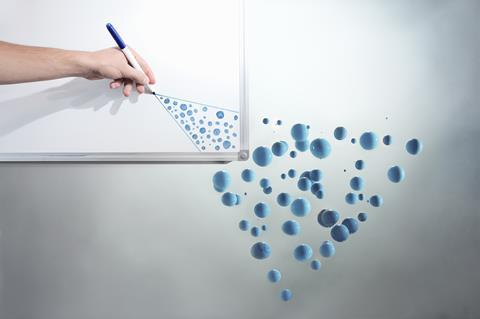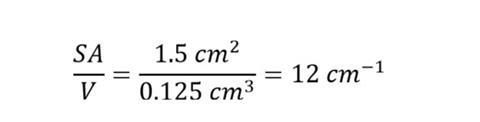A better grasp of geometric shapes will support students in their chemistry learningHelp students grasp geometry’s role in chemistry

Geometrical shapes are everywhere in chemistry. Before you carry on reading, grab a pen and paper or your smartphone and make a note of all the instances of shapes, angles, volumes and areas that students may encounter in pre-16 chemistry.

This article looks at just two aspects of chemistry – molecular structures and rates of reaction – and considers some of the geometrical concepts students use and how to support them to understand these ideas.

## Find out what they know

In pre-16 chemistry, students encounter various simple molecules, giant covalent structures – such as diamond and graphite – and buckyballs. An appreciation of polygons (2D shapes) and polyhedra (3D shapes) is essential to making sense of these molecular shapes, as well as explaining the properties of these compounds.

Learning chemistry can be challenging enough for some students without us assuming mathematical knowledge that they don’t have. Firstly, find out the following from your school’s maths department:

• What mathematical formulas and concepts are students meant to know by age 16? This will depend on specifications.
• When do students encounter these ideas during their secondary school years?
• How are these ideas taught? What terminology and formulas are used?

A diagnostic exercise and practice maths, algebra and chemistry problems as MS Word or pdf and answer sheet as MS Word or pdf.

A diagnostic exercise and practice maths, algebra and chemistry problems from the Education in Chemistry website: rsc.li/3jLyC6G

Students spend a considerable amount of time in their chemistry lessons looking at or drawing 2D representations of a 3D world. From states of matter to organic reaction mechanisms, the richness of three dimensions is often reduced to the plane of the page.

Your students’ spatial reasoning – the ability to think about an object in 3D and, in particular, create mental images – is likely to be varied, and you can support students by providing physical or 3D computational models for them to touch, rotate and flip. Some students may find some 3D models more helpful or engaging than others, so consider introducing a variety of approaches as described below.

Simple covalent molecules: valence shell electron pair repulsion (VSEPR) rules go beyond the scope of this article, but this simple balloon exercise can help familiarise students with molecular shapes. You can tie balloons at their nozzles to model linear, trigonal planar, tetrahedral, octahedral and even trigonal bipyramidal (PF5) bonding arrangements.

Graphite: you can illustrate graphite’s structure by asking students to place a carbon atom in the centre of a triangle and place three further carbon atoms at the vertices to slowly build a single graphite sheet. Once constructed, students should be able to calculate the bond angle (360°/3 = 120°) around each carbon. Although Molymod can be the obvious choice for many teachers, other construction toys – such as Geomag – are excellent alternatives.

Diamond: Ii diamond, carbon atoms form four covalent bonds to four other carbons in a tetrahedral arrangement. From maths, students may be more familiar with the term ‘triangular-based pyramid’ than tetrahedron, so bear this in mind. Students can visualise diamond’s structure as a carbon atom in the centre of a tetrahedron covalently bonded to four further carbon atoms at the vertices. Calculating the bond angles of 109.5° is not so simple, but your more able students may appreciate giving it a go or at least enjoy a written explanation.

Buckyballs: the buckyball structure is most easily visualised using Molymod – you need a lot of carbons – or using a computer model that you can rotate. Its C60 carbon frame gives rise to a structure composed of 20 hexagons and 12 pentagons. The article, Symmetry of buckminsterfullerene gives a more in-depth analysis of the mathematics and symmetry behind buckyballs.

## Surface area and volume in rates of reaction

An appreciation of surface area and volume is important in understanding reaction rates and the particular properties of nanoparticles. Whether discussing marble chips reacting with hydrochloric acid or nanoparticles, these particles will often be modelled as small cubes with a side of length l. One of the advantages of modelling particles as cubes is that each of the six sides is identical so calculating the surface area, volume and the ratio is simpler than for other 3D shapes.

## Calculating the surface area to volume ratio for a cube

Students need to follow four steps for this crucial calculation.

### 1. Calculating the surface area

Students need to be able to visualise the areas of each face of a 3D shape to calculate the surface area. For some students and some shapes, this will be straightforward. However, nets – showing an ‘unfolded’ 3D shape – can be a useful precursor for helping students who struggle with this. In the case of a cube, each of the six faces is identical, making this calculation simpler: 6 x l x l. It’s important for students to know that areas are expressed in m2, cm2, mm2 or nm2.

Take an example cube where l is 0.5 cm:

surface area = 6 x 0.5 x 0.5 = 1.5 cm2

### 2. Calculating the volume

A cube is simply a special case of a cuboid, where the volume is calculated as follows:

volume = height x width x length

In the case of the cube, all the lengths are the same:

volume = length x length length

volume = 0.5 x 0.5 0.5 = 0.125 cm3

### 3. Calculating the surface area to volume ratio

The surface area to volume ratio can be expressed as a ratio or, more commonly, the number (quotient) obtained by dividing the surface area by the volume.So for the example above, SA/V is:It’s important for students to know the units of cm-1 are a result of dividing an area in cm2 by a volume in cm3.

### 4. Comparing ratios

Calculating SA/V is one thing but understanding what it represents is another. SA/V is a way of stating that ‘for every one unit of volume, there are X many units of surface area’. When understood in a chemical context, reactants with a greater SA/V will lead to a greater rate of reaction (assuming all other variables are kept constant).

Here are few questions you might want to ask your students to probe their understanding:

• If my surface area doubles, what happens to the value of SA/V?
• If my volume doubles, what happens to the value of SA/V?
• I have two types of marble chip. One has a SA/V of 6 cm-1 and the other 0.6 cm-1. Which type of marble chip will react faster with hydrochloric acid?

## Build a strong foundation

There is no doubt a thorough familiarity with mathematical concepts goes a long way towards helping students to be better chemists and recognise the overlap between the two disciplines. In fact, you may be surprised by the number of maths exam questions at 16 that reference chemical concepts and it’s definitely worth exploring past maths papers to find a few gems. A strong foundation in geometry will prove invaluable for post-16 chemistry, underpinning transitional metal complexes, reaction mechanisms and molecular shapes among others.

Joe Ogborn is an independent science writer with experience in writing both educational and marketing communications.